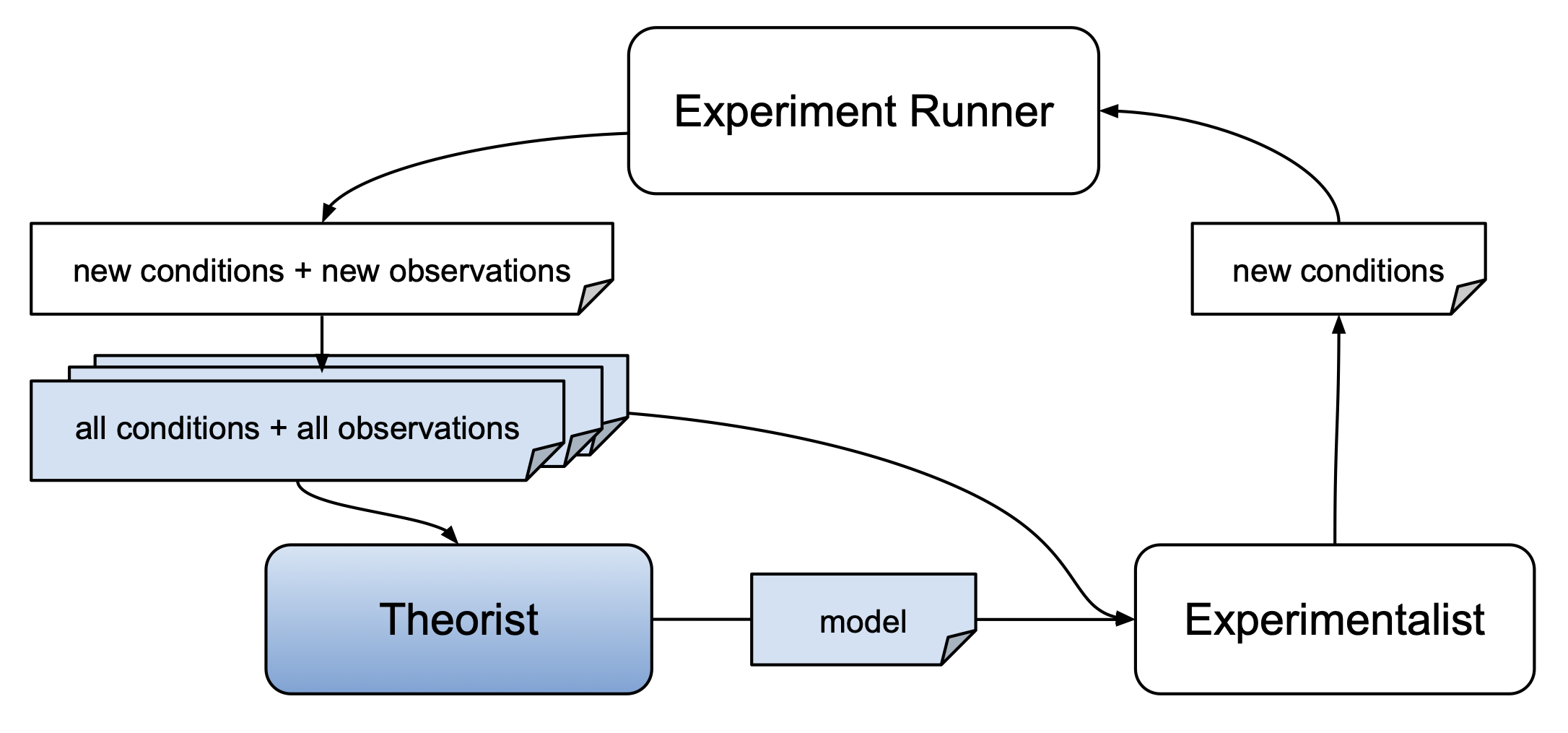# Theorist Overview

AutoRA consists of a set of techniques designed to automate the construction of interpretable models from data. To approach this problem, we can consider computational models as small, interpretable computation graphs (see also Musslick, 2021). A computation graph can take experiment parameters as input (e.g. the brightness of a visual stimulus) and can transform this input through a combination of functions to produce observable dependent measures as output (e.g. the probability that a participant can detect the stimulus).Theorist use information about experimental conditions that have already been probed $$\vec{x}' \in X'$$ and respective dependent measures $$\vec{y}' \in Y'$$. The following table includes the theorists currently implemented in AutoRA.

Bayesian Machine Scientist (BMS) Package, Docs A theorist that uses one algorithmic Bayesian approach to symbolic regression, with the aim of discovering interpretable expressions which capture relationships within data. $$X', Y'$$
Bayesian Symbolic Regression (BSR) Package, Docs A theorist that uses another algorithmic Bayesian approach to symbolic regression, with the aim of discovering interpretable expressions which capture relationships within data. $$X', Y'$$
Differentiable Architecture Search (DARTS) Package, Docs A theorist that automates the discovery of neural network architectures by making architecture search amenable to gradient descent. $$X', Y'$$